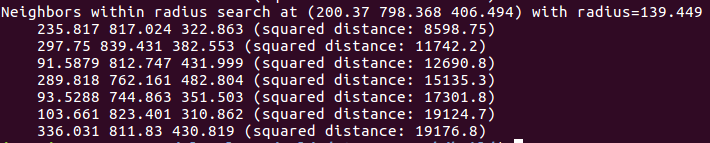# KD-tree 原理

kd树（k-dimensional树的简称），是一种分割k维数据空间的数据结构。主要应用于多维空间关键数据的搜索（如：范围搜索和最近邻搜索）。

Kd-tree的原理是这样的：不比较全部的k维数据，而是选择其中某一个维度比较，根据这个维度进行空间划分。

# 快速邻域搜索

## 求一个点的最紧邻点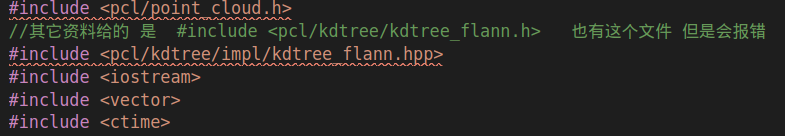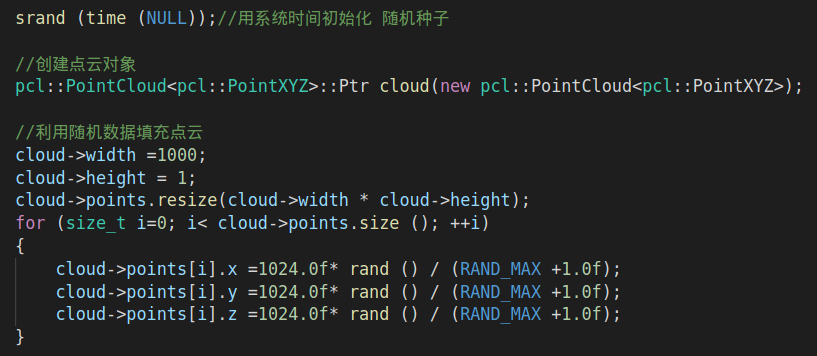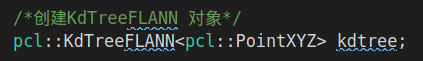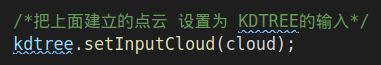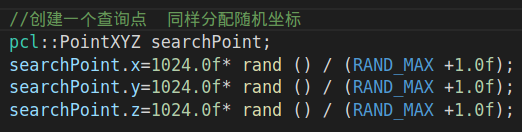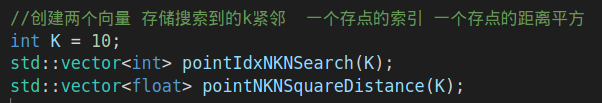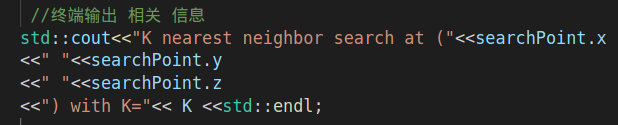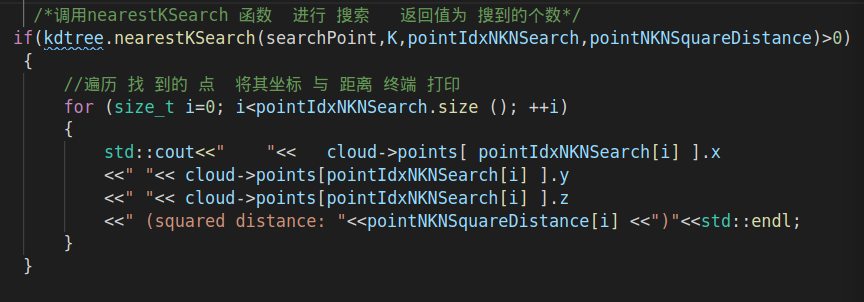1. 要查询的点
2. 查询返回的个数
3. 查询到的点的索引存放向量
4. 查询到的点的距离平方存放向量

### Code

#include <pcl/point_cloud.h>
//其它资料给的 是  #include <pcl/kdtree/kdtree_flann.h>   也有这个文件 但是会报错
#include <pcl/kdtree/impl/kdtree_flann.hpp>
#include <iostream>
#include <vector>
#include <ctime>

int main(int argc, char**argv)
{

srand (time (NULL));//用系统时间初始化 随机种子

//创建点云对象
pcl::PointCloud<pcl::PointXYZ>::Ptr cloud(new pcl::PointCloud<pcl::PointXYZ>);

//利用随机数据填充点云
cloud->width =1000;
cloud->height = 1;
cloud->points.resize(cloud->width * cloud->height);
for (size_t i=0; i< cloud->points.size (); ++i)
{
cloud->points[i].x =1024.0f* rand () / (RAND_MAX +1.0f);
cloud->points[i].y =1024.0f* rand () / (RAND_MAX +1.0f);
cloud->points[i].z =1024.0f* rand () / (RAND_MAX +1.0f);
}

/*创建KdTreeFLANN 对象*/
pcl::KdTreeFLANN<pcl::PointXYZ> kdtree;

/*把上面建立的点云 设置为 KDTREE的输入*/
kdtree.setInputCloud(cloud);

//创建一个查询点  同样分配随机坐标
pcl::PointXYZ searchPoint;
searchPoint.x=1024.0f* rand () / (RAND_MAX +1.0f);
searchPoint.y=1024.0f* rand () / (RAND_MAX +1.0f);
searchPoint.z=1024.0f* rand () / (RAND_MAX +1.0f);

//创建两个向量 存储搜索到的k紧邻  一个存点的索引 一个存点的距离平方
int K = 10;
std::vector<int> pointIdxNKNSearch(K);
std::vector<float> pointNKNSquareDistance(K);

//终端输出 相关 信息
std::cout<<"K nearest neighbor search at ("<<searchPoint.x
<<" "<<searchPoint.y
<<" "<<searchPoint.z
<<") with K="<< K <<std::endl;

/*调用nearestKSearch 函数  进行 搜索   返回值为 搜到的个数*/
if(kdtree.nearestKSearch(searchPoint,K,pointIdxNKNSearch,pointNKNSquareDistance)>0)
{
//遍历 找 到的 点  将其坐标 与 距离 终端 打印
for (size_t i=0; i<pointIdxNKNSearch.size (); ++i)
{
std::cout<<"    "<<   cloud->points[ pointIdxNKNSearch[i] ].x
<<" "<< cloud->points[pointIdxNKNSearch[i] ].y
<<" "<< cloud->points[pointIdxNKNSearch[i] ].z
<<" (squared distance: "<<pointNKNSquareDistance[i] <<")"<<std::endl;
}
}

return 0;
}


### Result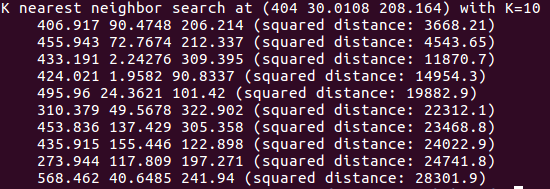## 求一个半径内的最紧邻点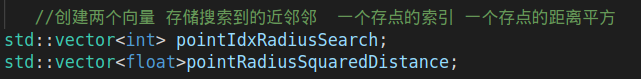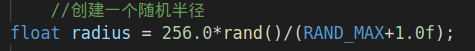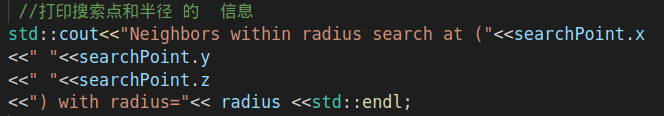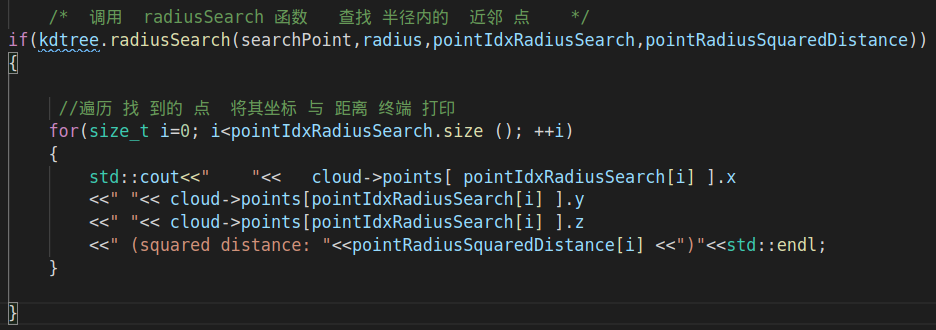1. 要查询的点
2. 查询的半径范围
3. 查询到的点的索引存放向量
4. 查询到的点的距离平方存放向量

### Code

       //创建两个向量 存储搜索到的近邻邻  一个存点的索引 一个存点的距离平方

//创建一个随机半径

//打印搜索点和半径 的  信息
std::cout<<"Neighbors within radius search at ("<<searchPoint.x
<<" "<<searchPoint.y
<<" "<<searchPoint.z

/*  调用  radiusSearch 函数   查找 半径内的  近邻 点    */
{

//遍历 找 到的 点  将其坐标 与 距离 终端 打印